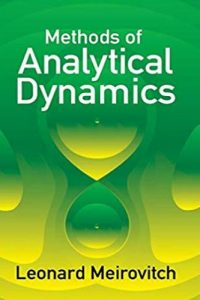اسم المؤلف
Leonard Meirovitch
التاريخ
24 أغسطس 2019
المشاهدات
التقييم(لا توجد تقييمات)Loading...

Methods of Analytical Dynamics
Leonard Meirovitch
Professor of Aerospace Engineering
University of Cincinnati
Contents
Preface vu
Chapter 1 Fundamentals of Newtonian Mechanics 1
1.1 Historical Survey of Mechanics
1.2 Newton’s Laws
1.3 Impulse and Momentum
1.4 Moment of a Force and Angular Momentum
1.5 Work and Energy
1.6 Energy Diagrams
1.7 Systems of Particles
1.8 The Two-Body Central Force Problem
1.9 The Inverse Square Law. Orbits of Planets and Satellites
1.10 Scattering by a Repulsive Central Force
Problems
Suggested References
Chapter 2 Fundamentals of Analytical Mechanics 45
2.1 Degrees of Freedom. Generalized Coordinates
2.2 Systems with Constraints
2.3 The Stationary Value of a Function
2.4 The Stationary Value of a Definite Integral
Contents
2.5 The Principle of Virtual Work
2.6 D’Alembert’s Principle
2.7 Hamilton’s Principle
2.8 Lagrange’s Equations of Motion
2.9 Lagrange’s Equations for Impulsive Forces
2.10 Conservation Laws
2.11 Routh’s Method for the Ignoration of Coordinates
2.12 Rayleigh’s Dissipation Function
2.13 Hamilton’s Equations
Problems
Suggested References
Chapter 3 Motion Relative to Rotating Reference Frames
3.1 Transformation of Coordinates
3.2 Rotating Coordinate Systems
3.3 Expressions for the Motion in Terms of Moving Reference Frames
3.4 Motion Relative to the Rotating Earth
3.5 Motion of a Free Particle Relative to the Rotating Earth
3.6 Foucault’s Pendulum
Problems
Suggested References
Chapter 4 Rigid Body Dynamics
4.1 Kinematics of a Rigid Body
4.2 The Linear and Angular Momentum of a Rigid Body
4.3 Translation Theorem for the Angular Momentum
4.4 The Kinetic Energy of a Rigid Body
4.5 Principal Axes
4.6 The Equations of Motion for a Rigid Body
4.7 Euler’s Equations of Motion
4.8 Euler’s Angles
4.9 Moment-Free Inertially Symmetric Body
4.10 General Case of a Moment-Free Body
4.11 Motion of a Symmetric Top
4.12 The Lagrangian Equations for Quasi-Coordinates
4.13 The Equations of Motion Referred to an Arbitrary System of Axes
4.14 The Rolling of a Coin
Problems
Suggested References
Chapter 5 Behavior of Dynamical Systems. Geometric Theory
5.1 Fundamental Concepts
5.2 Motion of Single-Degree-of-Freedom Autonomous Systems about
Equilibrium Points
178Contents xiii
5.3 Conservative Systems. Motion in the Large 189
5.4 The Index of Poincare
5.5 Limit Cycles of Poincare
Problems
Suggested References
Chapter 6 Stability of Multi-Degree-of-Freedom Autonomous Systems 209
6.1 General Linear Systems
6.2 Linear Autonomous Systems
6.3 Stability of Linear Autonomous Systems. Routh-Hurwitz
Criterion
6.4 The Variational Equations
6.5 Theorem on the First-Approximation Stability
6.6 Variation from Canonical Systems. Constant Coefficients
6.7 The Liapunov Direct Method
6.8 Geometric Interpretation of the Liapunov Direct Method
6.9 Stability of Canonical Systems
6.10 Stability in the Presence of Gyroscopic and Dissipative Forces
6.11 Construction of Liapunov Functions for Linear Autonomous Systems
Problems
Suggested References
Chapter 7 Nonautonomous Systems 263
7.1 Linear Systems with Periodic Coefficients. Floquet’s Theory
7.2 Stability of Variational Equations with Periodic Coefficients
7.3 Orbital Stability
7.4 Variation from Canonical Systems. Periodic Coefficients
7.5 Second-Order Systems with Periodic Coefficients
7.6 Hill’s Infinite Determinant
7.7 Mathieu’s Equation
7.8 The Liapunov Direct Method
Suggested References
Chapter 8 Analytical Solutions by Perturbation Techniques 293
8.1 The Fundamental Perturbation Technique
8.2 Secular Terms
8.3 Lindstedt’s Method
8.4 The Krylov-Bogoliubov-Mitropolsky (KBM) Method
8.5 A Perturbation Technique Based on Hill’s Determinants
8.6 Periodic Solutions of Nonautonomous Systems. Duffing’s Equation
8.7 The Method of Averaging
Problems
Suggested References
Contents
Chapter 9 Transformation Theory. The Hamilton-Jacobi Equation 329
9.1 The Principle of Least Action
9.2 Contact Transformations
9.3 Further Extensions of the Concept of Contact Transformations
9.4 Integral Invariants
9.5 The Lagrange and Poisson Brackets
9.6 Infinitesimal Contact Transformations
9.7 The Hamilton-Jacobi Equation
9.8 Separable Systems
9.9 Action and Angle Variables
9.10 Perturbation Theory
Problems
Suggested References
Chapter 10 The Gyroscope: Theory and Applications 381
10.1 Oscillations of a Symmetric Gyroscope
10.2 Effect of Gimbal Inertia on the Motion of a Free Gyroscope
10.3 Effect of Rotor Shaft Flexibility on the Frequency of Oscillation of
a Free Gyroscope
10.4 The Gyrocompass
10.5 The Gyropendulum. Schuler Tuning
10.6 Rate and Integrating Gyroscopes
Problems
Suggested References
Chapter 11 Problems in Celestial Mechanics
11.1 Kepler’s Equation. Orbit Determination
11.2 The Many-Body Problem
11.3 The Three-Body Problem
11.4 The Restricted Three-Body Problem
11.5 Stability of Motion Near the Lagrangian Points
11.6 The Equations of Relative Motion. Disturbing Function
11.7 Gravitational Potential and Torques for an Arbitrary Body
11.8 Precession and Nutation of the Earth’s Polar Axis
11.9 Variation of the Orbital Elements
11.10 The Resolution of the Disturbing Function
Problems
Suggested References
Chapter 12 Problems in Spacecraft Dynamics
12.1 Transfer Orbits. Changes in the Orbital Elements Due to a Small
Impulse
453Contents xv
12.2 Perturbations of a Satellite Orbit in the Gravitational Field of an
Oblate Planet
12.3 The Effect of Atmospheric Drag on Satellite Orbits
12.4 The Attitude Motion of Orbiting Satellites. General Considerations
12.5 The Attitude Stability of Earth-Pointing Satellites
12.6 The Attitude Stability of Spinning Symmetrical Satellites
12.7 Variable-Mass Systems
12.8 Rocket Dynamics
Problems
Suggested References
Appendix B Elements of Topology and Modern Analysis
B.l Sets and Functions
B.2 Metric Spaces
B.3 Topological Spaces
Suggested References
Name Index 507
Subject Index 511
كلمة سر فك الضغط : books-world.net